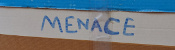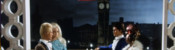mscroggs.co.ukmscroggs.co.uksubscribe

# Blog

## Proving a conjecture

2019-06-19
Last night at MathsJam, Peter Kagey showed me a conjecture about OEIS sequence A308092.A308092
The sum of the first $$n$$ terms of the sequence is the concatenation of the first $$n$$ bits of the sequence read as binary, with $$a(1) = 1$$.
1, 2, 3, 7, 14, 28, 56, 112, 224, 448, 896, 1791, 3583, 7166, ...To understand this definition, let's look at the first few terms of this sequence written in binary:
1, 10, 11, 111, 1110, 11100, 111000, 1110000, 11100000, 111000000, ...
By "the concatenation of the first $$n$$ bits of the sequence", it means the first $$n$$ binary digits of the whole sequence written in order: 1, then 11, then 110, then 1101, then 11011, then 110111, and so on. So the definition means:
• The first term is 1, as given in the definition ($$a(1)=1$$).
• The sum of the first 2 terms is the first 2 digits: $$1+10=11$$.
• The sum of the first 3 terms is the first 3 digits: $$1+10+11=110$$.
• The sum of the first 4 terms is the first 4 digits: $$1+10+11+111=1101$$.
• The sum of the first 5 terms is the first 5 digits: $$1+10+11+111+1110=11011$$.
As we know that the sum of the first $$n-1$$ terms is the first $$n-1$$ digits, we can calculate the third term of this sequence onwards using: "$$a(n)$$ is the concatenation of the first $$n$$ bits of the sequence subtract concatenation of the first $$n-1$$ bits of the sequence":
• The third term is $$110 - 11 = 11$$.
• The fourth term is $$1101 - 110 = 111$$.
• The fourth term is $$11011 - 1101 = 1110$$.
• The fifth term is $$110111 - 11011 = 11100$$.

### The conjecture

Peter's conjecture is that the number of 1s in each term is greater than or equal to the number of 1s in the previous term.
I'm going to prove this conjecture. If you'd like to have a try first, stop reading now and come back when you're ready for spoilers. (If you'd like a hint, read the next section then pause again.)

The third term of the sequence onwards can be calculated by subtracting the first $$n-1$$ digits from the first $$n$$ digits. If the first $$n-1$$ digits form a binary number $$x$$, then the first $$n$$ digits will be $$2x+d$$, where $$d$$ is the $$n$$th digit (because moving all the digits to the left one place in binary is multiplying by two).
Therefore the different is $$2x+d-x=x+d$$, and so we can work out the $$n$$th term of the sequence by adding the $$n$$th digit in the sequence to the first $$n-1$$ digits. (Hat tip to Martin Harris, who spotted this first.)

### Carrying

Adding 1 to a binary number the ends in 1 will cause 1 to carry over to the left. This carrying will continue until the 1 is carried into a position containing 0, and after this all the digits to the left of this 0 will remain unchanged.
Therefore adding a digit to the first $$n-1$$ digits can only change the digits from the rightmost 0 onwards.

### Endings

We can therefore disregard all the digits before the rightmost 0, and look at how the $$n$$th term compares to the $$(n-1)$$th term. There are 5 ways in which the first $$n$$ digits could end:
• $$00$$
• $$010$$
• $$01...10$$ (where $$1...1$$ is a string of 2 or more ones)
• $$01$$
• $$01...1$$ (where $$1...1$$ is again a string of 2 or more ones)
We now look at each of these in turn and show that the $$n$$th term will contain at least as many ones at the $$(n-1)$$th term.

#### Case 1: $$00$$

If the first $$n$$ digits of the sequence are $$x00$$ (a binary number $$x$$ followed by two zeros), then the $$(n-1)$$th term of the sequence is $$x+0=x$$, and the $$n$$th term of the sequence is $$x0+0=x0$$. Both $$x$$ and $$x0$$ contain the same number of ones.

#### Case 2: $$010$$

If the first $$n$$ digits of the sequence are $$x010$$, then the $$(n-1)$$th term of the sequence is $$x0+1=x1$$, and the $$n$$th term of the sequence is $$x01+0=x01$$. Both $$x1$$ and $$x01$$ contain the same number of ones.

#### Case 3: $$01...10$$

If the first $$n$$ digits of the sequence are $$x01...10$$, then the $$(n-1)$$th term of the sequence is $$x01...1+1=x10...0$$, and the $$n$$th term of the sequence is $$x01...10+1=x01...1$$. $$x01...1$$ contains more ones than $$x10...0$$.

#### Case 4: $$01$$

If the first $$n$$ digits of the sequence are $$x01$$, then the $$(n-1)$$th term of the sequence is $$x+0=x$$, and the $$n$$th term of the sequence is $$x0+1=x1$$. $$x1$$ contains one more one than $$x$$.

#### Case 5: $$01...1$$

If the first $$n$$ digits of the sequence are $$x01...1$$, then the $$(n-1)$$th term of the sequence is $$x01...1+1=x10...0$$, and the $$n$$th term of the sequence is $$x01...1+1=x10...0$$. Both these contain the same number of ones.

In all five cases, the $$n$$th term contains more ones or an equal number of ones to the $$(n-1)$$th term, and so the conjecture is true.

### Similar postsMathsteroidsBuilding MENACEs for other gamesBig Ben Strikes AgainChristmas (2016) is over

Comments in green were written by me. Comments in blue were not written by me.